# Teaching Calculus to a Deep Learner

Posted by Cleve Moler,

MIT's Professor Gil Strang gave two talks in one morning recently at the SIAM annual meeting. Both talks derived from his experience teaching a new course at MIT on linear algebra and neural nets. His first talk, "The Structure of a Deep Neural Net", was in a minisymposium titled "Deep Learning and Deep Teaching", which he organized. Another talk in that minisymposium was by Drexel's Professor Pavel Grinfeld on "An Informal Approach to Teaching Calculus." An hour later, Gil's gave his second talk, "Teaching About Learning." It was an invited talk at the SIAM Conference on Applied Mathematics Education.

Inspired by Pavel's talk about teaching calculus, Gil began his second talk with some spontaneous remarks. "Can we teach a deep learner calculus?" he wondered. The system might be trained with samples of functions and their derivatives and then be asked to find derivatives of other functions that were not the training set.

Immediately after Gil's second talk, I asked the other MathWorkers attending the meeting if we could take up Gil's challenge. Within a few hours, Mary Fenelon, Christine Tobler and Razvan Carbunescu had the essential portions of the following demonstration of the Neural Networks Toolbox® working at the MathWorks booth.

### Contents

#### Functions

Our first task is less ambitious than differentiation. It is simply to recognize the shapes of functions. By a function we mean the MATLAB vector obtained by sampling a familiar elementary function at a finite set of ordered random points drawn uniformly from the interval $[-1, 1]$. Derivatives, which we have not done yet, would be divided differences. We use six functions, $x$, $x^2$, $x^3$, $x^4$, $\sin{\pi x}$, and $\cos{\pi x}$. We attach a random sign and add white noise to the samples.

   F = {@(x) x, @(x) x.^2, @(x) x.^3, @(x) x.^4, ...
@(x) sin(pi*x), @(x) cos(pi*x)};
labels = ["x", "x^2", "x^3", "x^4", "sin(pi*x)", "cos(pi*x)"];


#### Definitions

Here are a few definitions and parameters.

Set random number generator state.

   rng(2)


Generate uniform random variable on [-1, 1].

   randu = @(m,n) (2*rand(m,n)-1);


Generate random +1 or -1.

   randsign = @() sign(randu(1,1));


Number of functions.

   m = length(F);


Number of repetitions.

   n = 1000;


Number of samples in the interval.

   nx = 100;


Noise level.

   noise = .0001;


#### Generate training set

C = cell(m,n);
for j = 1:n
x = sort(randu(nx,1));
for i = 1:m
C{i,j} = randsign()*F{i}(x) + noise*randn(nx,1);
end
end


#### Representive curves

Let's plot instance one of each function. (With this initialization of rng, the $x^2$ and $sin(\pi x)$ curves have negative signs.)

    set(gcf,'position',[300 300 300 300])
for i = 1:m
plot(x,C{i,1},'.')
axis([-1 1 -1 1])
title(labels(i))
snapnow
end
close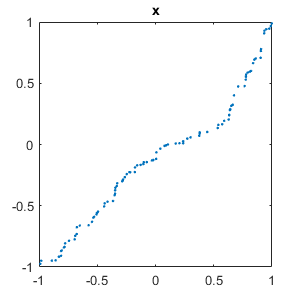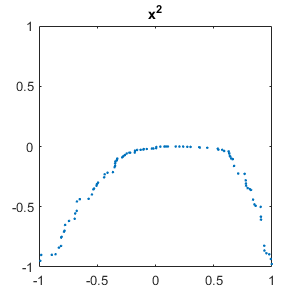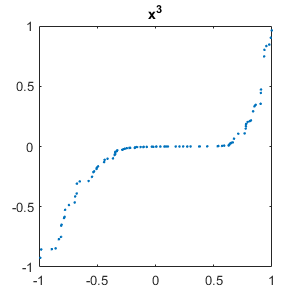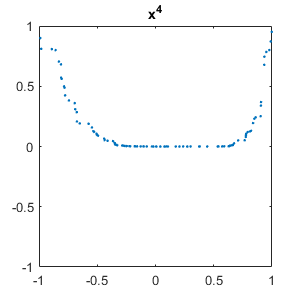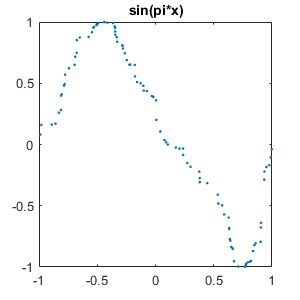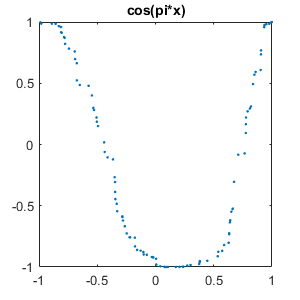#### Neural network layers

Our deep learning network is one that has proved to be successful in signal processing, text, and other applications with sequential data. There are six layers. The nonlinear activation layer, relu, for REctified Linear Unit, is essential. ReLU(x) is simply max(0,x). LSTM stands for Long Short-Term Memory. Softmax is a generalization of the logistic function used to compute probabilities.

    inputSize = nx;
numClasses = m;
numHiddenUnits = 100;
layers = [ ...
sequenceInputLayer(inputSize)
reluLayer
lstmLayer(numHiddenUnits,'OutputMode','last')
fullyConnectedLayer(numClasses)
softmaxLayer
classificationLayer];


#### Neural network options

The first option, 'adam', is the stochastic optimization algorithm, adaptive moment estimation. An epoch is one forward pass and one backward pass over all of the training vectors, updating the weights. In our experience with this network, six passes is enough.

    maxEpochs = 6;
miniBatchSize = 27;
'ExecutionEnvironment','cpu', ...
'MaxEpochs',maxEpochs, ...
'MiniBatchSize',miniBatchSize, ...
'Verbose',0, ...
'Plots','training-progress');


#### Train network

With our setting of the Plots option, trainNetwork opens a custom figure window that dynamically shows the progress of the optimation.

It takes 15 or 20 seconds to train this network on my laptop. Big time neural nets with more layers and more epochs can make use of GPUs and pools of parallel workers

    C = reshape(C',1,[]);
Y = repelem(categorical(labels'), n);
net = trainNetwork(C,Y,layers,options);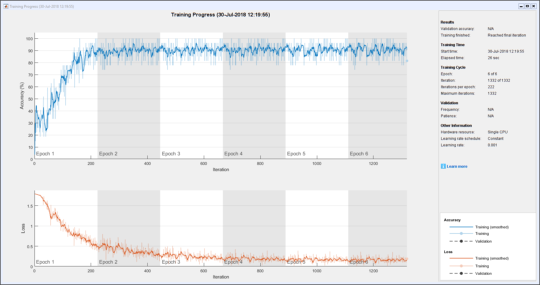#### Generate test set

Generate more functions to form a test set.

    nt = 100;
Ctest = cell(m,nt);
for j = 1:nt
x = sort(randu(nx,1));
for i = 1:m
Ctest{i,j} = randsign()*F{i}(x) + noise*randn(nx,1);
end
end


#### Classify

Classify the functions in the test set.

    miniBatchSize = 27;
Ctest = reshape(Ctest',1,[]);
Ytest = repelem(categorical(labels'), nt);
Ypred = classify(net,Ctest,'MiniBatchSize',miniBatchSize);


#### Plot results

Here are the results. We see scores above 95 percent, except for learning to distinguish between plots of $x^2$ and $x^4$. That's understandable.

    T = table(Ypred, Ytest);
heatmap(T, 'Ypred', 'Ytest');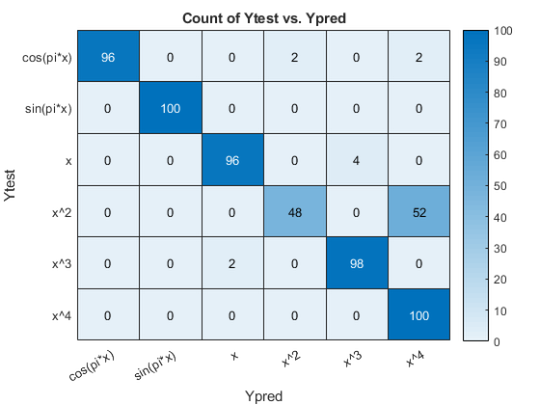#### Typical mismatches

Here are typical failed tests. It's not hard to see why the network is having trouble with these.

    set(gcf,'position',[300 300 300 300])
for j = 1:m
for i = [1:j-1 j+1:m]
mismatch = find(Ytest == labels(i) & Ypred == labels(j));
if ~isempty(mismatch)
% Plot one of each type of mismatch
for k = 1
plot(linspace(-1, 1), Ctest{mismatch(k)},'.')
title(labels(i)+" or "+labels(j))
snapnow
end
end
end
end
close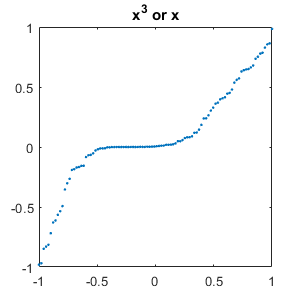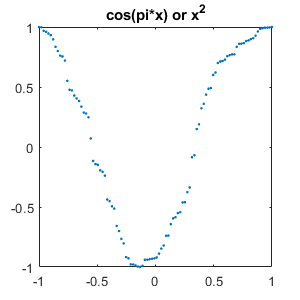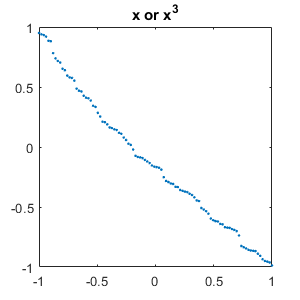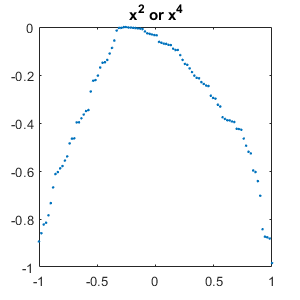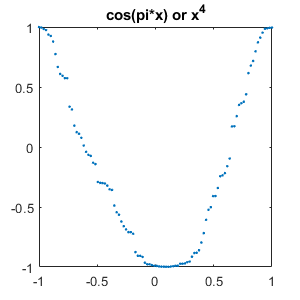#### Thanks

Thanks to my colleagues attending the SIAM meeting -- Mary, Christine and Razvan. And thanks to Gil for his spontaneous idea.

Get the MATLAB code

Published with MATLAB® R2018a